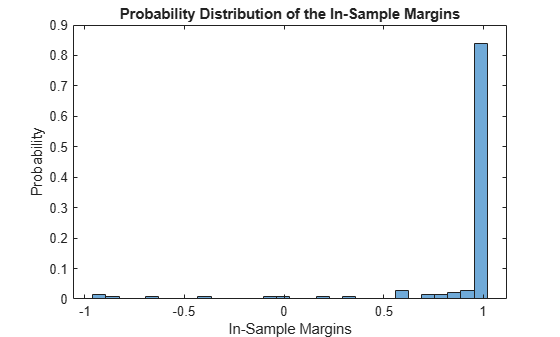# resubMargin

Resubstitution classification margins for naive Bayes classifier

## Syntax

``m = resubMargin(Mdl)``

## Description

example

````m = resubMargin(Mdl)` returns the resubstitution Classification Margin (`m`) for the naive Bayes classifier `Mdl` using the training data stored in `Mdl.X` and the corresponding class labels stored in `Mdl.Y`.`m` is returned as a numeric vector with the same length as `Y`. The software estimates each entry of `m` using the trained naive Bayes classifier `Mdl`, the corresponding row of `X`, and the true class label `Y`.```

## Examples

collapse all

Estimate the resubstitution (in-sample) classification margins of a naive Bayes classifier. An observation margin is the observed true class score minus the maximum false class score among all scores in the respective class.

Load the `fisheriris` data set. Create `X` as a numeric matrix that contains four petal measurements for 150 irises. Create `Y` as a cell array of character vectors that contains the corresponding iris species.

```load fisheriris X = meas; Y = species;```

Train a naive Bayes classifier using the predictors `X` and class labels `Y`. A recommended practice is to specify the class names. `fitcnb` assumes that each predictor is conditionally and normally distributed.

`Mdl = fitcnb(X,Y,'ClassNames',{'setosa','versicolor','virginica'})`
```Mdl = ClassificationNaiveBayes ResponseName: 'Y' CategoricalPredictors: [] ClassNames: {'setosa' 'versicolor' 'virginica'} ScoreTransform: 'none' NumObservations: 150 DistributionNames: {'normal' 'normal' 'normal' 'normal'} DistributionParameters: {3x4 cell} Properties, Methods ```

`Mdl` is a trained `ClassificationNaiveBayes` classifier.

Estimate the resubstitution classification margins.

```m = resubMargin(Mdl); median(m)```
```ans = 1.0000 ```

Display the histogram of the in-sample classification margins.

```histogram(m,30,'Normalization','probability') xlabel('In-Sample Margins') ylabel('Probability') title('Probability Distribution of the In-Sample Margins')```Classifiers that yield relatively large margins are preferred.

Perform feature selection by comparing in-sample margins from multiple models. Based solely on this comparison, the model with the highest margins is the best model.

Load the `fisheriris` data set. Specify the predictors `X` and class labels `Y`.

```load fisheriris X = meas; Y = species;```

Define these two data sets:

• `fullX` contains all predictors.

• `partX` contains the last two predictors.

```fullX = X; partX = X(:,3:4);```

Train a naive Bayes classifier for each predictor set.

```FullMdl = fitcnb(fullX,Y); PartMdl = fitcnb(partX,Y);```

Estimate the in-sample margins for each classifier.

```fullM = resubMargin(FullMdl); median(fullM)```
```ans = 1.0000 ```
```partM = resubMargin(PartMdl); median(partM)```
```ans = 1.0000 ```

The two models have similar performance. However, `PartMdl` is less complex.

## Input Arguments

collapse all

Full, trained naive Bayes classifier, specified as a `ClassificationNaiveBayes` model trained by `fitcnb`.

collapse all

### Classification Edge

The classification edge is the weighted mean of the classification margins.

If you supply weights, then the software normalizes them to sum to the prior probability of their respective class. The software uses the normalized weights to compute the weighted mean.

When choosing among multiple classifiers to perform a task such as feature section, choose the classifier that yields the highest edge.

### Classification Margin

The classification margin for each observation is the difference between the score for the true class and the maximal score for the false classes. Margins provide a classification confidence measure; among multiple classifiers, those that yield larger margins (on the same scale) are better.

### Posterior Probability

The posterior probability is the probability that an observation belongs in a particular class, given the data.

For naive Bayes, the posterior probability that a classification is k for a given observation (x1,...,xP) is

`$\stackrel{^}{P}\left(Y=k|{x}_{1},..,{x}_{P}\right)=\frac{P\left({X}_{1},...,{X}_{P}|y=k\right)\pi \left(Y=k\right)}{P\left({X}_{1},...,{X}_{P}\right)},$`

where:

• $P\left({X}_{1},...,{X}_{P}|y=k\right)$ is the conditional joint density of the predictors given they are in class k. `Mdl.DistributionNames` stores the distribution names of the predictors.

• π(Y = k) is the class prior probability distribution. `Mdl.Prior` stores the prior distribution.

• $P\left({X}_{1},..,{X}_{P}\right)$ is the joint density of the predictors. The classes are discrete, so $P\left({X}_{1},...,{X}_{P}\right)=\sum _{k=1}^{K}P\left({X}_{1},...,{X}_{P}|y=k\right)\pi \left(Y=k\right).$

### Prior Probability

The prior probability of a class is the assumed relative frequency with which observations from that class occur in a population.

### Classification Score

The naive Bayes score is the class posterior probability given the observation.